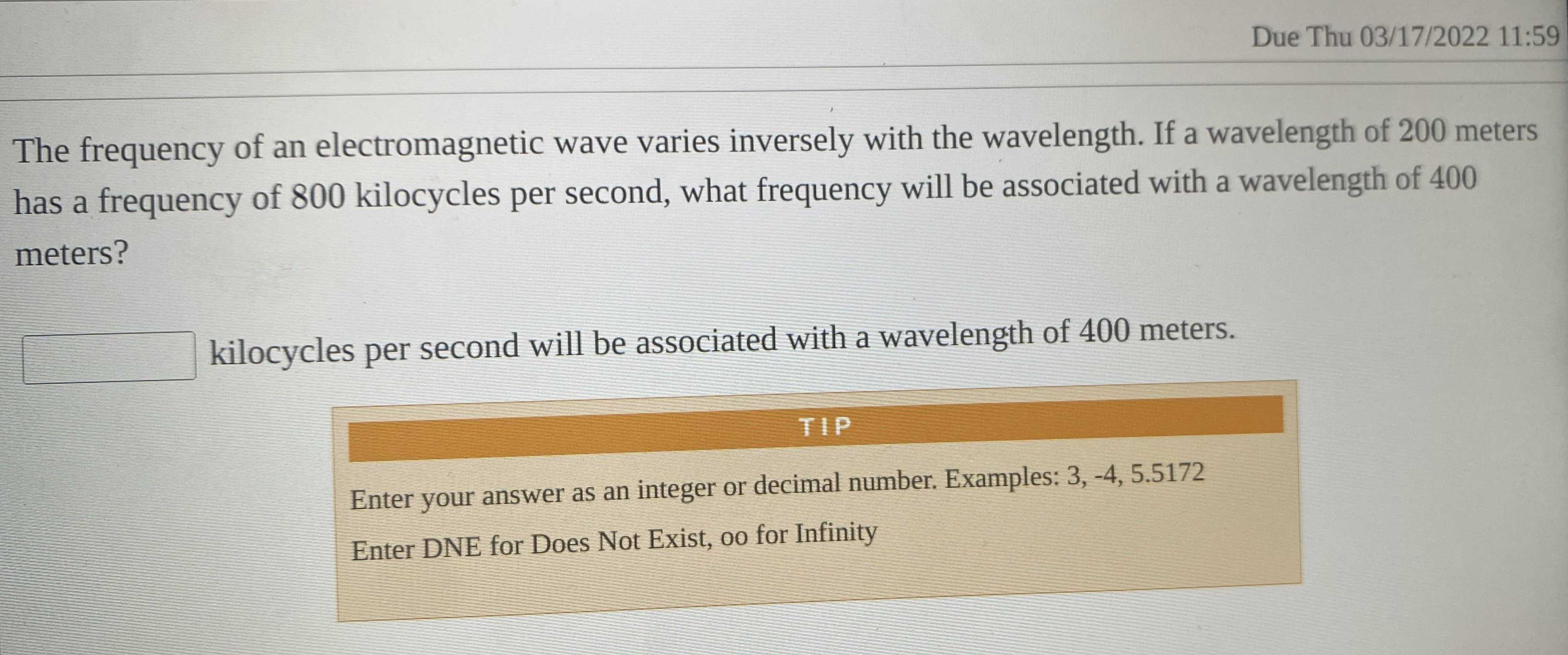### Still have math questions?

Algebra
QuestionThe frequency of an electromagnetic wave varies inversely with the wavelength. If a wavelength of $$200$$ meters  has a frequency of $$800$$ kilocycles per second, what frequency will be associated with a wavelength of $$400$$  meters?

kilocycles per second will be associated with a wavelength of $$400$$ meters.

Enter your answer as an integer or decimal number. Examples: $$3 , - 4,5.5172$$

Enter DNE for Does Not Exist, oo for Infinity